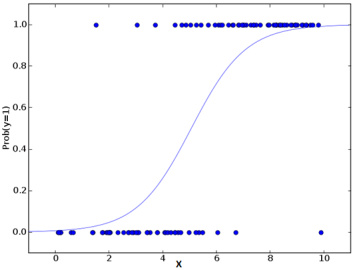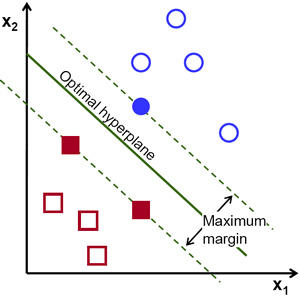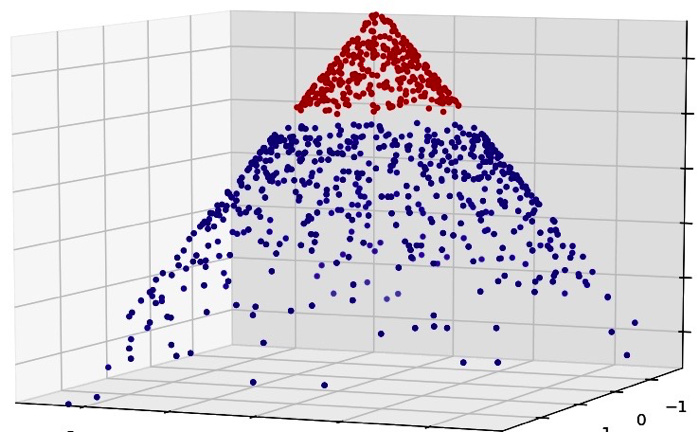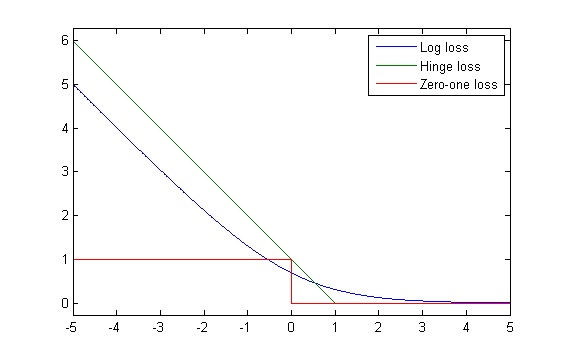# 1. 两种算法的思路比较SVM 试图找出一个超平面，模型优化的目标是使距离它最近的点到它的距离之和最大，通常通过核技巧使线性不可分的数据集映射到新的特征空间。# 2. 参数估计方式

LR 实际上是对线性回归模型的预测结果取逼近真实标记的对数几率（log odds），即反映了预测结果接近真实的可能性，可能性当然是越大越好。用最大似然估计法来估计参数 $w$，它是一种参数估计方法，LR的损失函数形式如下

\begin{equation}
J(\theta)=-\frac{1}{m}\sum_{i=1}^{m}[y^{(i)}logh_w(x^{(i)})+(1-y^{(i)})log(1-h_w(x^{(i)}))]
\end{equation}

\begin{equation}
L(w,b,\alpha)=\frac{1}{2}{||w||}^2-\sum_{i=1}^m{\alpha_i(1-y_i(w^Tx_i+b))} \label{lagrangefunction}
\end{equation}# 3. 实验效果比较

## 3.1 单变量分布情形

1. 一般情况下，多项式核 SVM 的误分类率比较高。另外，LR和线性核、径向基核、sigmoid 核 SVM 模型的表现差不多。当样本容量发生变化，sigmoid 核和多项式核比其他核的误分类率更低
2. 样本数据不平衡的时候，LR 比 SVM 更胜一筹
3. 在样本服从指数分布情形下，除了多项式核，SVM 跟 LR 表现得一样好，样本容量分布不平衡时，LR 比 SVM 模型表现更好；样本服从正态分布、泊松分布时，不推荐多项式核 SVM## 3.2 混合分布情形

1. 正态-泊松混合分布：SVM 比 LR 表现要好，特别是 d 很小

2. 柯西-正态混合分布：SVM 模型比 LR 表现更佳

# 4. 小结

1. 视具体情况而定，优先考虑 LR，LR 简单、快速、易于解释，实在不行再考虑 SVM
2. LR 得到的是一个可以解释决策置信度的概率，它的目标函数没有约束条件，并且十分平滑，而 SVM 不是基于概率的，它的目标函数有约束条件，因此两者的参数估计手段完全不同
3. SVM 的泛化性能佳，SVM 不会对有足够置信度的点支付一个惩罚，所以假设一个数据集已经被 SVM 求解，那么将一类点删除或是增加并不会改变 SVM 的求解结果
4. LR 在不平衡数据集中的表现优于 SVM，SVM 在样本服从混合分布情形下表现优于 LR
5. LR 和 SVM 对数据的敏感程度不同，LR 中每一个数据点都会影响到效果，而 SVM 的性能并不依赖于整体数据
6. SVM 需要考虑数据的几何距离和函数距离，所以处理时需要对数据做归一化处理，而 LR 则不需要

# 5. 讨论

@梁斌penny: svm核方法升维，使得在高维上的线性切割，投射到实际空间就变为非线性的切割；lr通过增加大量非线性特征，使得获得非线性切割能力；深度学习通过二层以上神经网络获得非线性切割。核心都需要有非线性识别能力

@phunter_lau: 其实解决问题最有效的模型是LR和SVM，然后是决策树系列xgboost，对于特定结构化数据才是深度学习，最近火是因为一些之前不能做的大规模结构化数据问题能搞了，这不能说其他模型就没用了对吧

# 6. 推荐阅读

1. http://www.kurims.kyoto-u.ac.jp/EMIS/journals/RCE/V35/v35n2a03.pdf